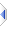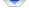# round

 Definition: atom x2 = round(atom x1, atom inverted_precision=1) Description: round x1 to the specified precision. Comments: The precision is the inverse of what you might have expected, for example: to round a number to the nearest 0.1, specify an inverted precision of 10, to round a number to the nearest 1000, specify an inverted precision of 0.001. This function may be applied to an atom or sq_round() to all elements of a sequence. The rules for sequence operations apply. Example: ```r = round(1.4) -- r is 1 r = round(1.6) -- r is 2 r = round(8.136,100) -- r is 8.14 ``` Implementation: See builtins\pmaths.e (an autoinclude) for details of the actual implementation.